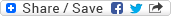## Monday, May 26, 2008

### Train, Tunnel, and Man. How faster is the train running?

Puzzle: A man has entered into a tunnel and has crossed only 1/4 of it while it hears a whistle from a train behind him. He turns and runs towards the train with the same speed and he could barely get out of tunnel before the train (running with a constant speed) could hit him at the entrance of the tunnel. Had he moved in the same direction with the same speed then also he could have crossed the tunnel before the train could have hit him at the exit of the tunnel. Assume that the speed of the man is uniform, he takes zero time to turn back, and instantly gets his speed while turning back. How faster is the train moving as compared to the man?

Solution: Let the speed of the train be X and that of the man be Y. Let the length of the tunnel be T and the distance of the train from the entrance of the tunnel at the time the man turned back is E.

Now, we can easily form two euqations with the given data. One, The man covered T/4 distance and the train covered E distance in the same time, hence

E/X = (T/4)/Y

=> X/Y = E/(T/4)

=> X/Y = 4E/T ..... (i)

Two, The man could have covered 3T/4 distance and the train could have covered E + T in the same time, hence

(E+T)/X = (3T/4)/Y

=> X/Y = (E+T)/(3T/4)

=> X/Y = 4(E+T)/3T ..... (ii)

Comparing (i) & (ii), we get

4E/T = 4(E+T)/3T

=> E = (E+T)/3

=> 3E = E + T

=> T = 2E

putting this value in equation (i), we get

X/Y = 4E/2E

=> X/Y = 2

That means the train is running twice as fast as the man.